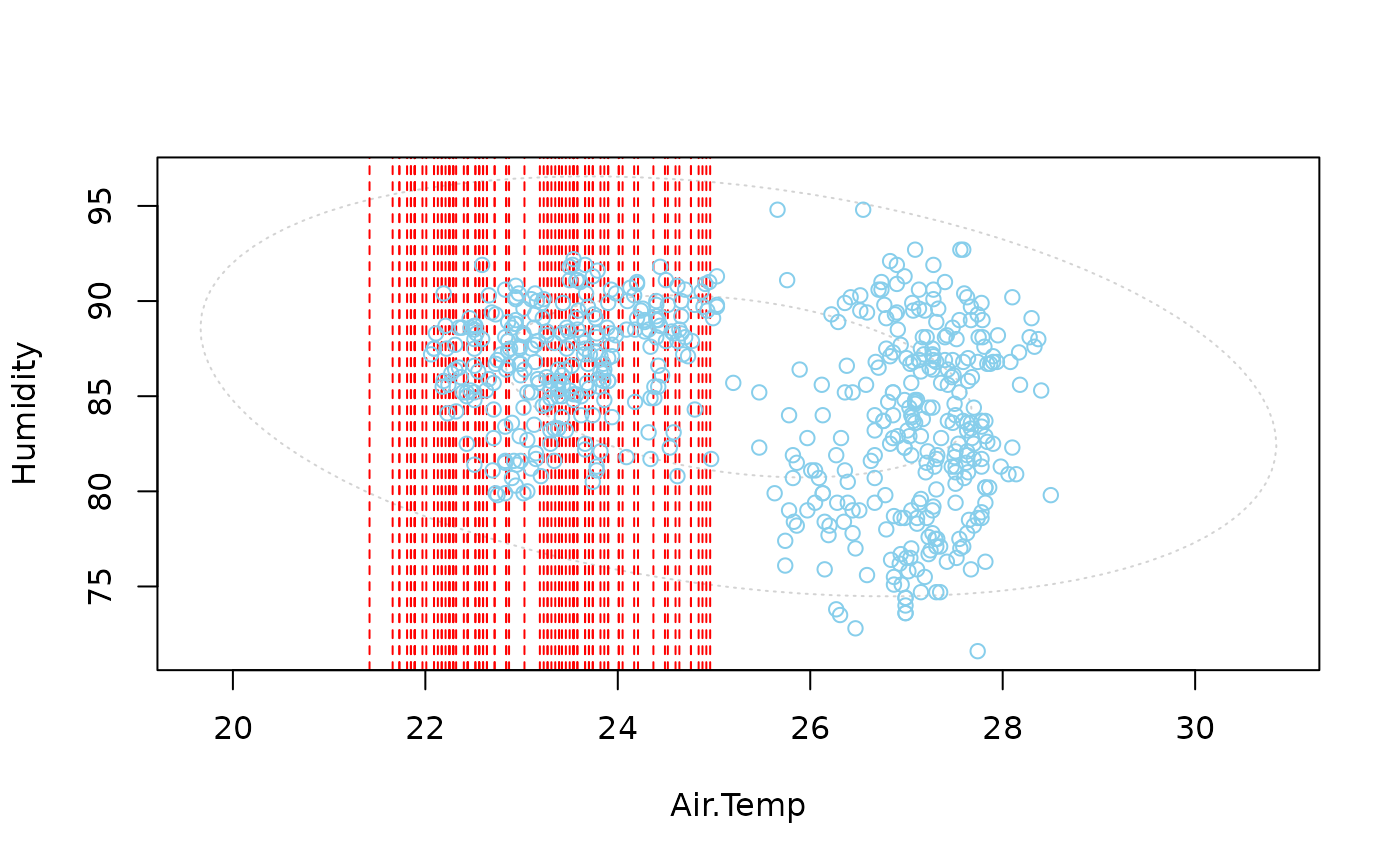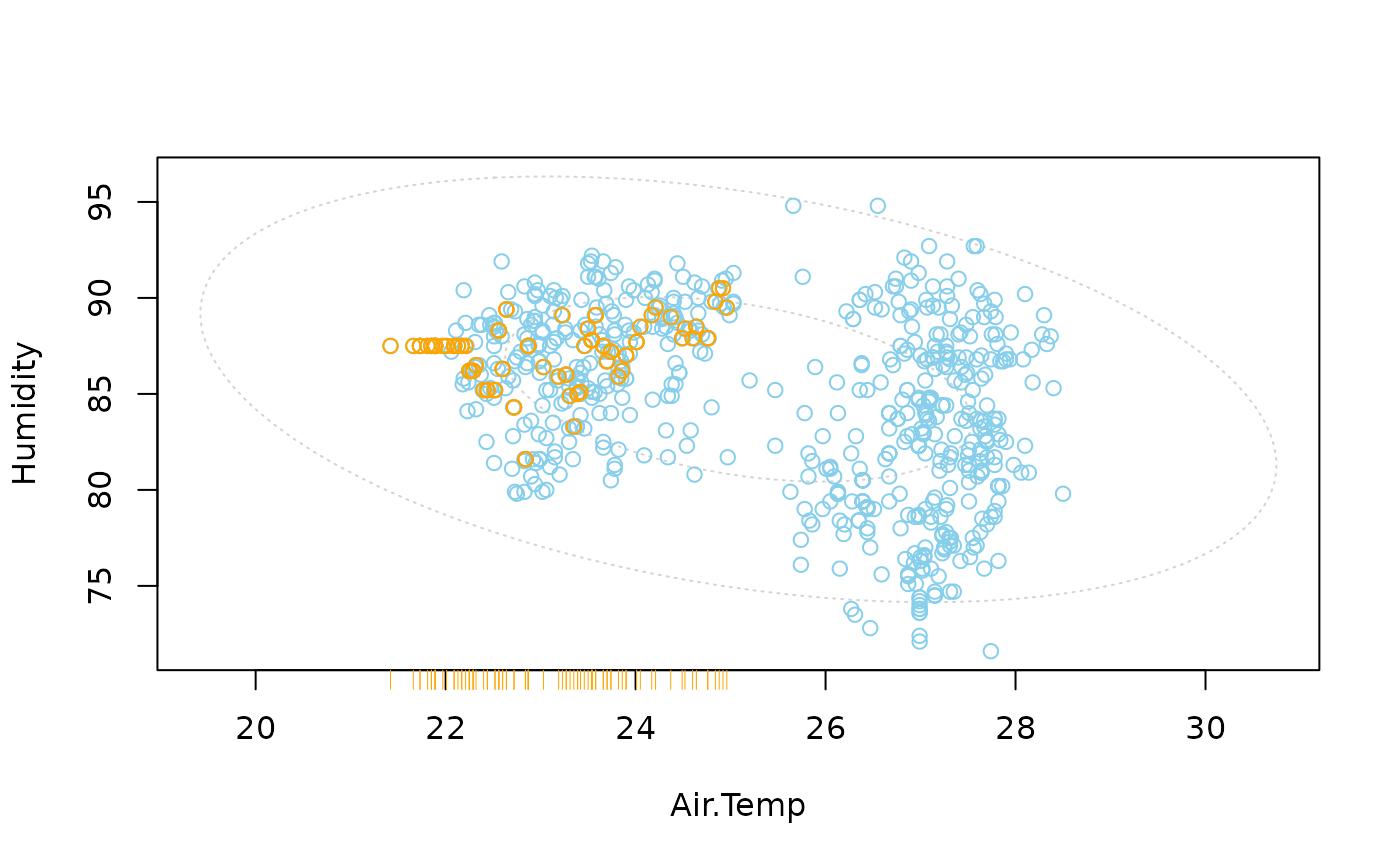In addition to a standard scatterplot, lines are plotted for the missing values in one variable. If there are imputed values, they will be highlighted.

scattMiss(
x,
delimiter = NULL,
side = 1,
col = c("skyblue", "red", "orange", "lightgrey"),
alpha = NULL,
lty = c("dashed", "dotted"),
lwd = par("lwd"),
quantiles = c(0.5, 0.975),
inEllipse = FALSE,
zeros = FALSE,
xlim = NULL,
ylim = NULL,
main = NULL,
sub = NULL,
xlab = NULL,
ylab = NULL,
interactive = TRUE,
...
)

## Arguments

x

a matrix or data.frame with two columns.

delimiter

a character-vector to distinguish between variables and imputation-indices for imputed variables (therefore, x needs to have colnames()). If given, it is used to determine the corresponding imputation-index for any imputed variable (a logical-vector indicating which values of the variable have been imputed). If such imputation-indices are found, they are used for highlighting and the colors are adjusted according to the given colors for imputed variables (see col).

side

if side=1, a rug representation and vertical lines are plotted for the missing/imputed values in the second variable; if side=2, a rug representation and horizontal lines for the missing/imputed values in the first variable.

col

a vector of length four giving the colors to be used in the plot. The first color is used for the scatterplot, the second/third color for the rug representation for missing/imputed values. The second color is also used for the lines for missing values. Imputed values will be highlighted with the third color, and the fourth color is used for the ellipses (see ‘Details’). If only one color is supplied, it is used for the scatterplot, the rug representation and the lines, whereas the default color is used for the ellipses. Else if a vector of length two is supplied, the default color is used for the ellipses as well.

alpha

a numeric value between 0 and 1 giving the level of transparency of the colors, or NULL. This can be used to prevent overplotting.

lty

a vector of length two giving the line types for the lines and ellipses. If a single value is supplied, it will be used for both.

lwd

a vector of length two giving the line widths for the lines and ellipses. If a single value is supplied, it will be used for both.

quantiles

a vector giving the quantiles of the chi-square distribution to be used for the tolerance ellipses, or NULL to suppress plotting ellipses (see ‘Details’).

inEllipse

plot lines only inside the largest ellipse. Ignored if quantiles is NULL or if there are imputed values.

zeros

a logical vector of length two indicating whether the variables are semi-continuous, i.e., contain a considerable amount of zeros. If TRUE, only the non-zero observations are used for computing the tolerance ellipses. If a single logical is supplied, it is recycled. Ignored if quantiles is NULL.

xlim, ylim

axis limits.

main, sub

main and sub title.

xlab, ylab

axis labels.

interactive

a logical indicating whether the side argument can be changed interactively (see ‘Details’).

...

further graphical parameters to be passed down (see graphics::par()).

## Details

Information about missing values in one variable is included as vertical or horizontal lines, as determined by the side argument. The lines are thereby drawn at the observed x- or y-value. In case of imputed values, they will additionally be highlighted in the scatterplot. Supplementary, percentage coverage ellipses can be drawn to give a clue about the shape of the bivariate data distribution.

If interactiveis TRUE, clicking in the bottom margin redraws the plot with information about missing/imputed values in the first variable and clicking in the left margin redraws the plot with information about missing/imputed values in the second variable. Clicking anywhere else in the plot quits the interactive session.

## Note

The argument zeros has been introduced in version 1.4. As a result, some of the argument positions have changed.

marginplot()

Other plotting functions: aggr(), barMiss(), histMiss(), marginmatrix(), marginplot(), matrixplot(), mosaicMiss(), pairsVIM(), parcoordMiss(), pbox(), scattJitt(), scattmatrixMiss(), spineMiss()

## Author

Andreas Alfons, modifications by Bernd Prantner

## Examples


data(tao, package = "VIM")
## for missing values
scattMiss(tao[,c("Air.Temp", "Humidity")])## for imputed values
scattMiss(kNN(tao[,c("Air.Temp", "Humidity")]), delimiter = "_imp")